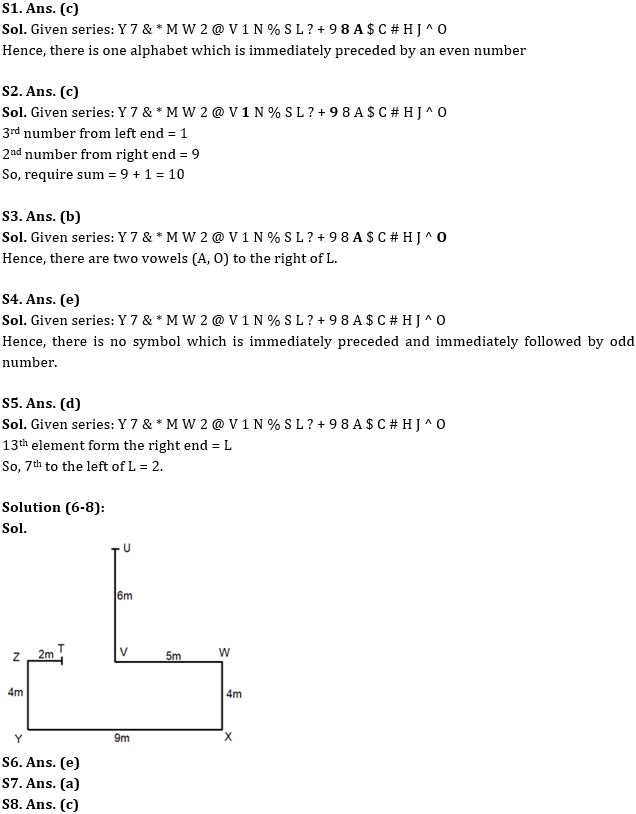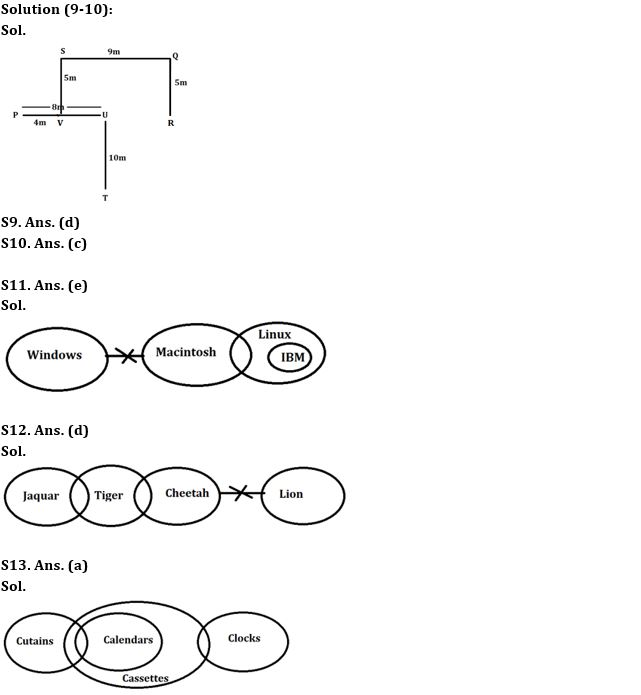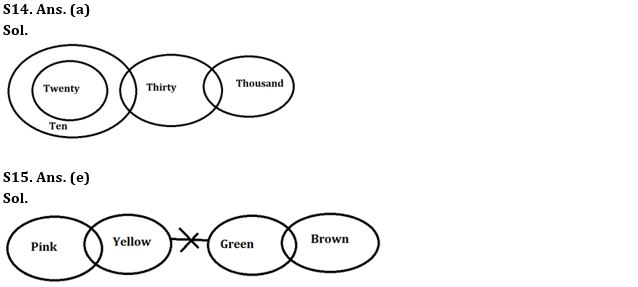Latest Banking jobs   »

# Reasoning Ability Quiz For IBPS Clerk/PO Prelims 2022- 9th August

Directions (1-5): Study the following arrangement carefully and answer the questions

Y 7 & * M W 2 @ V 1 N % S L ? + 9 8 A \$ C # H J ^ O

Q1. How many alphabets are immediately preceded by an even number?
(a) None
(b) Two
(c) One
(d) Three
(e) More than three

Q2. What is the sum of 3rd number from left end and 2nd number from right end?
(a) 19
(b) 13
(c) 10
(d) 12
(e) 18

Q3. How many vowels are right to the L?
(a) None
(b) Two
(c) One
(d) Three
(e) More than three

Q4. How many symbols are immediately preceded and immediately followed by odd number?
(a) One
(b) Two
(c) Three
(d) Four
(e) None of the above

Q5. Which of the following element is 7th to the left of 13th element from right end?
(a) V
(b) @
(c) W
(d) 2
(e) None of these

Directions (6-8): Answer the questions based on the information given below.
Nitin started walking from point U in south direction. After walking 6m he turns left from point V and walks for 5m till point W. He then turns right and walks for 4m till point X and then turns right and walks for 9m till point Y. He then turns right and walks for 4m till point Z and then turns right and walks for 2m till point T.

Q6. What is the direction of T with respect to W?
(a) North-east
(b) North-west
(c) South-east
(d) South-west
(e) West

Q7. What is the direction of U with respect to Y?
(a) North-east
(b) North-west
(c) South
(d) South-west
(e) West

Q8. What is the shortest distance between point T and W?
(a) 6m
(b) 9m
(c) 7m
(d) 10m
(e) None of these

Directions (9-10): Answer the questions based on the information given below.
Seven points P, Q, R, S, T, U and V are drawn on sheet of paper. S is 5m north of V. T is 10m south of U. Q is 5m north of R. P is 8m west of U. V is 4m east of P. Q is 9m east of S.

Q9. What is the shortest distance between P and R?
(a) 10m
(b) 11m
(c) 12m
(d) 13m
(e) None of these

Q10. What is the direction of T with respect to S?
(a) North-east
(b) North-west
(c) South-east
(d) South-west
(e) None of these

Directions (11-15): In the question below, three statements are given followed by the conclusions. You have to take the given statements to be true even if they seem to be at variance with commonly known facts. Read all the conclusions and then decide which of the given conclusions logically follows from the given statements disregarding commonly known facts.

Q11. Statements: No Window is Macintosh.
A few Macintosh are Linux.
Only Linux are IBM
Conclusion: I. Some Macintosh is definitely IBM
II. Some IBM can be Windows
(a) Only conclusion I follows
(b) Only conclusion II follows
(c) Either conclusion I or II follows
(d) Both conclusions I and II follow
(e) Neither conclusion I nor II follows

Q12. Statements: No Cheetah is Lion.
Only a few Cheetah are Tiger.
Mostly tiger are Jaquar.
Conclusions: I. All Cheetah can be Jaquar
II. Some Lion can be Jaquar
(a) Only conclusion I follows
(b) Only conclusion II follows
(c) Either conclusion I or II follows
(d) Both conclusions I and II follow
(e) Neither conclusion I nor II follows

Q13. Statements: Some curtains are calendar.
All calendars are cassettes.
Some cassettes are clocks.
Conclusions: I. Some cassettes are curtains
II. All curtains are cassettes
(a) Only conclusion I follows
(b) Only conclusion II follows
(c) Either conclusion I or II follows
(d) Both conclusions I and II follow
(e) Neither conclusion I nor II follows

Q14. Statements: Only ten are twenty.
Mostly ten are thirty.
Some thirty are thousand
Conclusions: I. No twenty is thousand
II. Some thirty being twenty is a possibility
(a) Only conclusion I follows
(b) Only conclusion II follows
(c) Either conclusion I or II follows
(d) Both conclusions I and II follow
(e) Neither conclusion I nor II follows

Q15. Statements: Only a few pink are yellow.
No yellow is green.
Mostly green are brown
Conclusions: I. Some pink are not brown
II. All pink being yellow is a possibility
(a) Only conclusion I follows
(b) Only conclusion II follows
(c) Either conclusion I or II follows
(d) Both conclusions I and II follow
(e) Neither conclusion I nor II follows

Solutions#### Congratulations!Download Hindu Review of October 2021: Free PDF